# LECTURE 1. Control Systems Engineering_MEB 4101.pdf

24 May 2023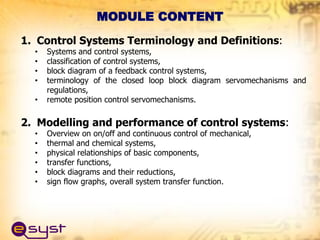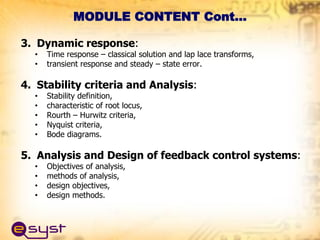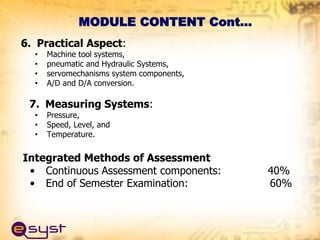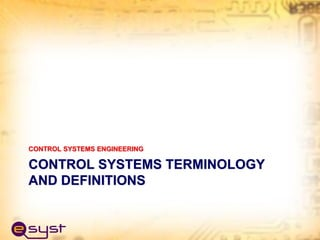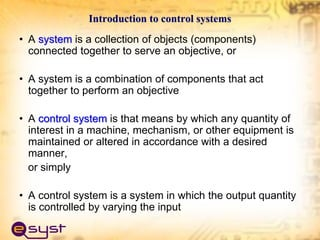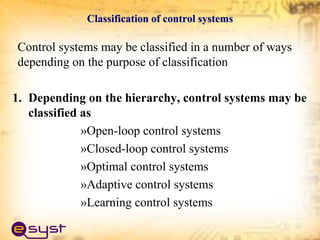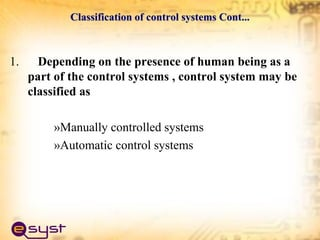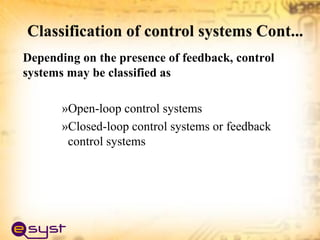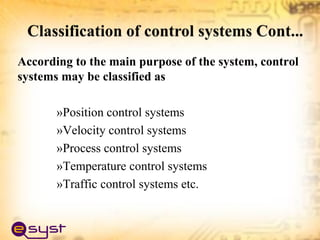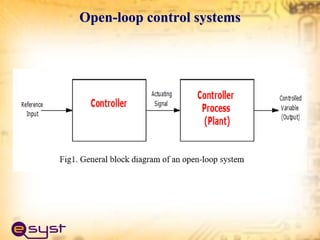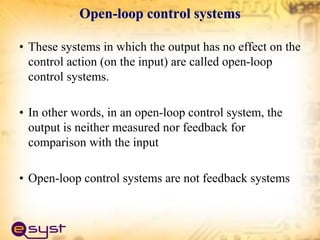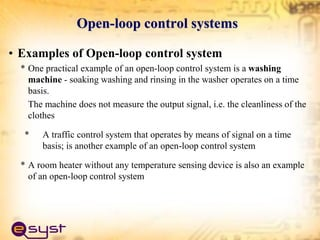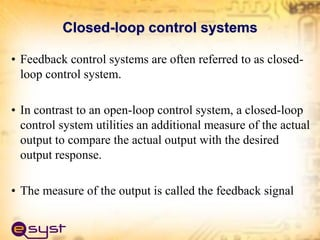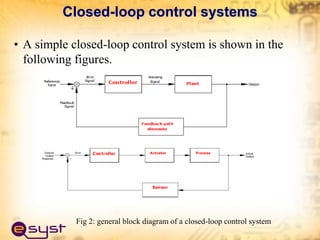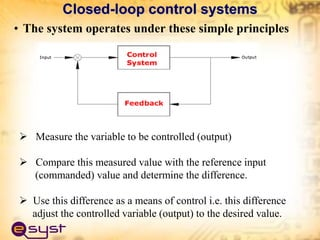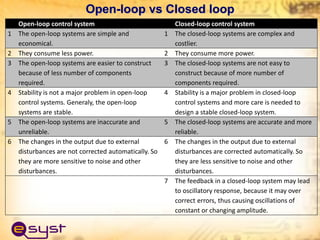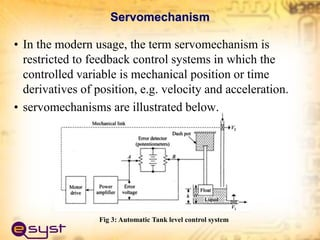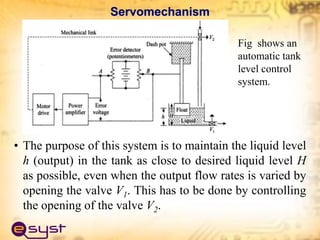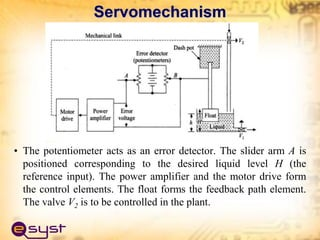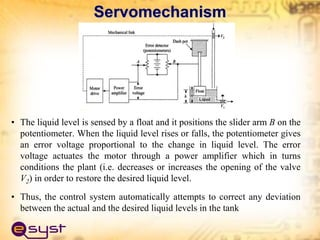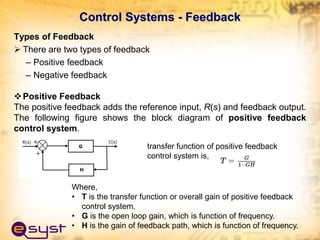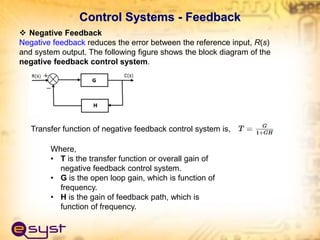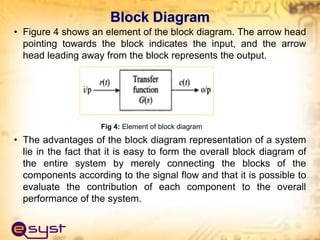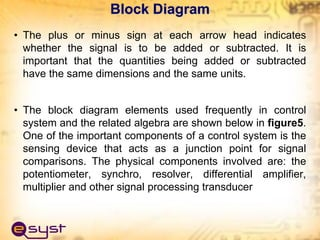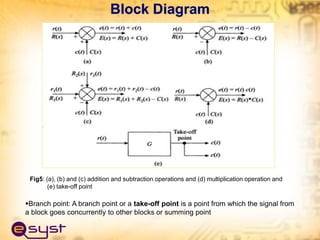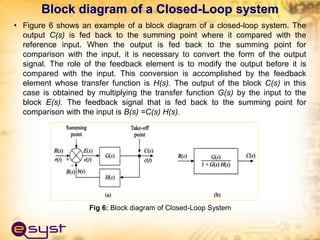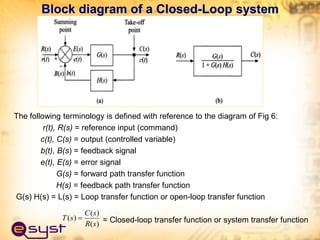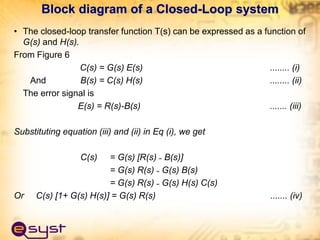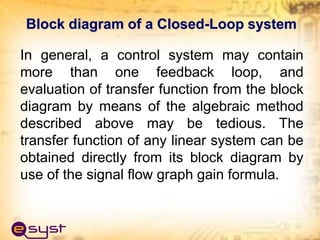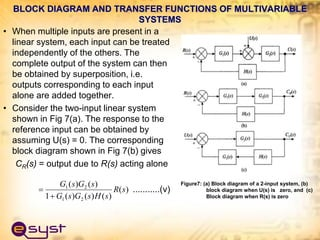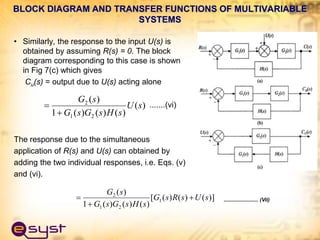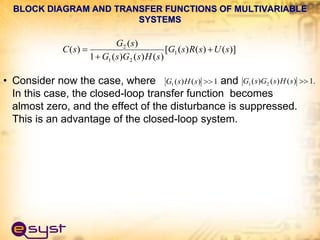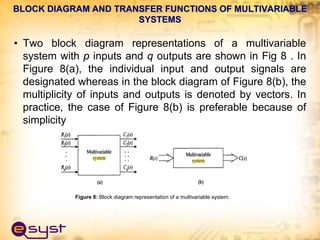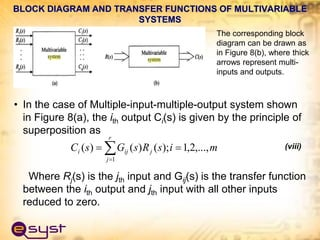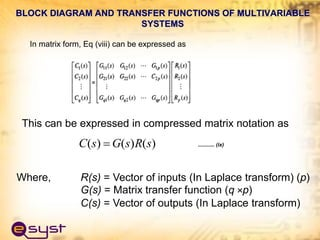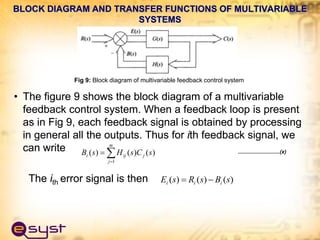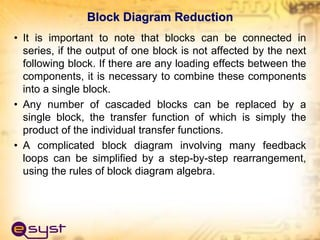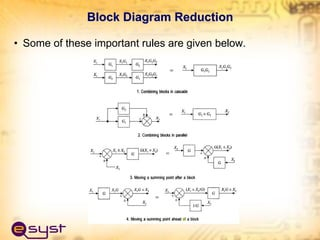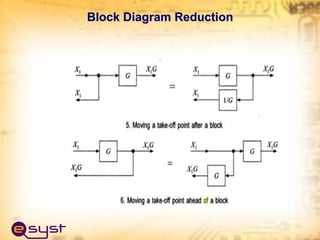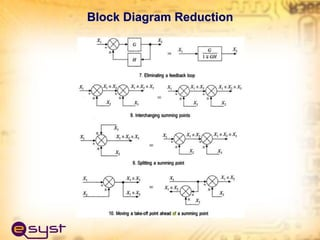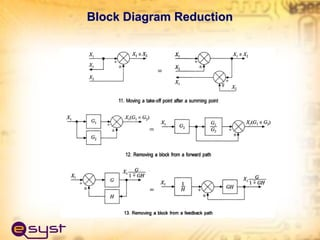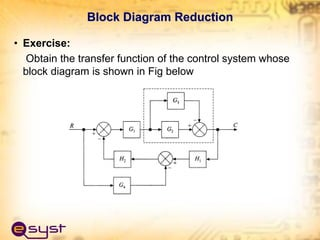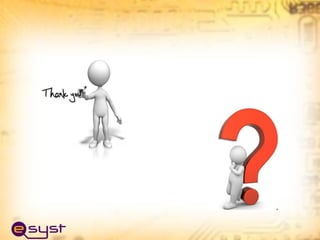1 sur 44

### LECTURE 1. Control Systems Engineering_MEB 4101.pdf

• 1. CONTROL SYSTEMS ENGINEERING Course Code: MEB 4101 Course Credit 4 Introduction Prepared by; Masoud Kamoleka Mlela BSc, Electromechanical Eng. (UDSM) MSc, Renewable Energy (UDSM) PhD, Mechanical Engineering (HEU, CN).
• 2. 1. Control Systems Terminology and Definitions: • Systems and control systems, • classification of control systems, • block diagram of a feedback control systems, • terminology of the closed loop block diagram servomechanisms and regulations, • remote position control servomechanisms. 2. Modelling and performance of control systems: • Overview on on/off and continuous control of mechanical, • thermal and chemical systems, • physical relationships of basic components, • transfer functions, • block diagrams and their reductions, • sign flow graphs, overall system transfer function. MODULE CONTENT
• 3. 3. Dynamic response: • Time response – classical solution and lap lace transforms, • transient response and steady – state error. 4. Stability criteria and Analysis: • Stability definition, • characteristic of root locus, • Rourth – Hurwitz criteria, • Nyquist criteria, • Bode diagrams. 5. Analysis and Design of feedback control systems: • Objectives of analysis, • methods of analysis, • design objectives, • design methods. MODULE CONTENT Cont…
• 4. 6. Practical Aspect: • Machine tool systems, • pneumatic and Hydraulic Systems, • servomechanisms system components, • A/D and D/A conversion. 7. Measuring Systems: • Pressure, • Speed, Level, and • Temperature. Integrated Methods of Assessment • Continuous Assessment components: 40% • End of Semester Examination: 60% MODULE CONTENT Cont…
• 5. CONTROL SYSTEMS TERMINOLOGY AND DEFINITIONS CONTROL SYSTEMS ENGINEERING
• 6. Introduction to control systems • A system is a collection of objects (components) connected together to serve an objective, or • A system is a combination of components that act together to perform an objective • A control system is that means by which any quantity of interest in a machine, mechanism, or other equipment is maintained or altered in accordance with a desired manner, or simply • A control system is a system in which the output quantity is controlled by varying the input
• 7. Classification of control systems Control systems may be classified in a number of ways depending on the purpose of classification 1. Depending on the hierarchy, control systems may be classified as »Open-loop control systems »Closed-loop control systems »Optimal control systems »Adaptive control systems »Learning control systems
• 8. Classification of control systems Cont... 1. Depending on the presence of human being as a part of the control systems , control system may be classified as »Manually controlled systems »Automatic control systems
• 9. Depending on the presence of feedback, control systems may be classified as »Open-loop control systems »Closed-loop control systems or feedback control systems
• 10. According to the main purpose of the system, control systems may be classified as »Position control systems »Velocity control systems »Process control systems »Temperature control systems »Traffic control systems etc.
• 11. Open-loop control systems
• 12. Open-loop control systems • These systems in which the output has no effect on the control action (on the input) are called open-loop control systems. • In other words, in an open-loop control system, the output is neither measured nor feedback for comparison with the input • Open-loop control systems are not feedback systems
• 13. Open-loop control systems • Examples of Open-loop control system * One practical example of an open-loop control system is a washing machine - soaking washing and rinsing in the washer operates on a time basis. The machine does not measure the output signal, i.e. the cleanliness of the clothes * A traffic control system that operates by means of signal on a time basis; is another example of an open-loop control system * A room heater without any temperature sensing device is also an example of an open-loop control system
• 14. Closed-loop control systems • Feedback control systems are often referred to as closed- loop control system. • In contrast to an open-loop control system, a closed-loop control system utilities an additional measure of the actual output to compare the actual output with the desired output response. • The measure of the output is called the feedback signal
• 15. • A simple closed-loop control system is shown in the following figures. Closed-loop control systems Fig 2: general block diagram of a closed-loop control system
• 16. • The system operates under these simple principles  Measure the variable to be controlled (output)  Compare this measured value with the reference input (commanded) value and determine the difference.  Use this difference as a means of control i.e. this difference adjust the controlled variable (output) to the desired value. Closed-loop control systems
• 17. Open-loop vs Closed loop Open-loop control system Closed-loop control system 1 The open-loop systems are simple and economical. 1 The closed-loop systems are complex and costlier. 2 They consume less power. 2 They consume more power. 3 The open-loop systems are easier to construct because of less number of components required. 3 The closed-loop systems are not easy to construct because of more number of components required. 4 Stability is not a major problem in open-loop control systems. Generaly, the open-loop systems are stable. 4 Stability is a major problem in closed-loop control systems and more care is needed to design a stable closed-loop system. 5 The open-loop systems are inaccurate and unreliable. 5 The closed-loop systems are accurate and more reliable. 6 The changes in the output due to external disturbances are not corrected automatically. So they are more sensitive to noise and other disturbances. 6 The changes in the output due to external disturbances are corrected automatically. So they are less sensitive to noise and other disturbances. 7 The feedback in a closed-loop system may lead to oscillatory response, because it may over correct errors, thus causing oscillations of constant or changing amplitude.
• 18. Servomechanism • In the modern usage, the term servomechanism is restricted to feedback control systems in which the controlled variable is mechanical position or time derivatives of position, e.g. velocity and acceleration. • servomechanisms are illustrated below. Fig 3: Automatic Tank level control system
• 19. Servomechanism • The purpose of this system is to maintain the liquid level h (output) in the tank as close to desired liquid level H as possible, even when the output flow rates is varied by opening the valve V1. This has to be done by controlling the opening of the valve V2. Fig shows an automatic tank level control system.
• 20. • The potentiometer acts as an error detector. The slider arm A is positioned corresponding to the desired liquid level H (the reference input). The power amplifier and the motor drive form the control elements. The float forms the feedback path element. The valve V2 is to be controlled in the plant. Servomechanism
• 21. • The liquid level is sensed by a float and it positions the slider arm B on the potentiometer. When the liquid level rises or falls, the potentiometer gives an error voltage proportional to the change in liquid level. The error voltage actuates the motor through a power amplifier which in turns conditions the plant (i.e. decreases or increases the opening of the valve V2) in order to restore the desired liquid level. • Thus, the control system automatically attempts to correct any deviation between the actual and the desired liquid levels in the tank Servomechanism
• 22. Control Systems - Feedback Types of Feedback  There are two types of feedback – Positive feedback – Negative feedback Positive Feedback The positive feedback adds the reference input, R(s) and feedback output. The following figure shows the block diagram of positive feedback control system. transfer function of positive feedback control system is, Where, • T is the transfer function or overall gain of positive feedback control system. • G is the open loop gain, which is function of frequency. • H is the gain of feedback path, which is function of frequency.
• 23.  Negative Feedback Negative feedback reduces the error between the reference input, R(s) and system output. The following figure shows the block diagram of the negative feedback control system. Transfer function of negative feedback control system is, Where, • T is the transfer function or overall gain of negative feedback control system. • G is the open loop gain, which is function of frequency. • H is the gain of feedback path, which is function of frequency. Control Systems - Feedback
• 24. Block Diagram • Figure 4 shows an element of the block diagram. The arrow head pointing towards the block indicates the input, and the arrow head leading away from the block represents the output. Fig 4: Element of block diagram • The advantages of the block diagram representation of a system lie in the fact that it is easy to form the overall block diagram of the entire system by merely connecting the blocks of the components according to the signal flow and that it is possible to evaluate the contribution of each component to the overall performance of the system.
• 25. • The plus or minus sign at each arrow head indicates whether the signal is to be added or subtracted. It is important that the quantities being added or subtracted have the same dimensions and the same units. • The block diagram elements used frequently in control system and the related algebra are shown below in figure5. One of the important components of a control system is the sensing device that acts as a junction point for signal comparisons. The physical components involved are: the potentiometer, synchro, resolver, differential amplifier, multiplier and other signal processing transducer Block Diagram
• 26. Block Diagram Fig5: (a), (b) and (c) addition and subtraction operations and (d) multiplication operation and (e) take-off point Branch point: A branch point or a take-off point is a point from which the signal from a block goes concurrently to other blocks or summing point
• 27. • Figure 6 shows an example of a block diagram of a closed-loop system. The output C(s) is fed back to the summing point where it compared with the reference input. When the output is fed back to the summing point for comparison with the input, it is necessary to convert the form of the output signal. The role of the feedback element is to modify the output before it is compared with the input. This conversion is accomplished by the feedback element whose transfer function is H(s). The output of the block C(s) in this case is obtained by multiplying the transfer function G(s) by the input to the block E(s). The feedback signal that is fed back to the summing point for comparison with the input is B(s) =C(s) H(s). Block diagram of a Closed-Loop system Fig 6: Block diagram of Closed-Loop System
• 28. The following terminology is defined with reference to the diagram of Fig 6: r(t), R(s) = reference input (command) c(t), C(s) = output (controlled variable) b(t), B(s) = feedback signal e(t), E(s) = error signal G(s) = forward path transfer function H(s) = feedback path transfer function G(s) H(s) = L(s) = Loop transfer function or open-loop transfer function = Closed-loop transfer function or system transfer function Block diagram of a Closed-Loop system ) ( ) ( ) ( s R s C s T 
• 29. • The closed-loop transfer function T(s) can be expressed as a function of G(s) and H(s). From Figure 6 C(s) = G(s) E(s) ........ (i) And B(s) = C(s) H(s) ........ (ii) The error signal is E(s) = R(s)-B(s) ....... (iii) Substituting equation (iii) and (ii) in Eq (i), we get C(s) = G(s) [R(s) ˗ B(s)] = G(s) R(s) ˗ G(s) B(s) = G(s) R(s) ˗ G(s) H(s) C(s) Or C(s) [1+ G(s) H(s)] = G(s) R(s) ....... (iv) Block diagram of a Closed-Loop system
• 30. In general, a control system may contain more than one feedback loop, and evaluation of transfer function from the block diagram by means of the algebraic method described above may be tedious. The transfer function of any linear system can be obtained directly from its block diagram by use of the signal flow graph gain formula. Block diagram of a Closed-Loop system
• 31. BLOCK DIAGRAM AND TRANSFER FUNCTIONS OF MULTIVARIABLE SYSTEMS • When multiple inputs are present in a linear system, each input can be treated independently of the others. The complete output of the system can then be obtained by superposition, i.e. outputs corresponding to each input alone are added together. • Consider the two-input linear system shown in Fig 7(a). The response to the reference input can be obtained by assuming U(s) = 0. The corresponding block diagram shown in Fig 7(b) gives CR(s) = output due to R(s) acting alone ...........(v) Figure7: (a) Block diagram of a 2-input system, (b) block diagram when U(s) is zero, and (c) Block diagram when R(s) is zero ) ( ) ( ) ( ) ( 1 ) ( ) ( 2 1 2 1 s R s H s G s G s G s G  
• 32. • Similarly, the response to the input U(s) is obtained by assuming R(s) = 0. The block diagram corresponding to this case is shown in Fig 7(c) which gives Cu(s) = output due to U(s) acting alone .......(vi) The response due to the simultaneous application of R(s) and U(s) can obtained by adding the two individual responses, i.e. Eqs. (v) and (vi). ) ( ) ( ) ( ) ( 1 ) ( 2 1 2 s U s H s G s G s G   )] ( ) ( ) ( [ ) ( ) ( ) ( 1 ) ( 1 2 1 2 s U s R s G s H s G s G s G    ....................... (Vii) BLOCK DIAGRAM AND TRANSFER FUNCTIONS OF MULTIVARIABLE SYSTEMS
• 33. • Consider now the case, where and In this case, the closed-loop transfer function becomes almost zero, and the effect of the disturbance is suppressed. This is an advantage of the closed-loop system. 1 ) ( ) ( 1  s H s G . 1 ) ( ) ( ) ( 2 1  s H s G s G )] ( ) ( ) ( [ ) ( ) ( ) ( 1 ) ( ) ( 1 2 1 2 s U s R s G s H s G s G s G s C    BLOCK DIAGRAM AND TRANSFER FUNCTIONS OF MULTIVARIABLE SYSTEMS
• 34. • Two block diagram representations of a multivariable system with p inputs and q outputs are shown in Fig 8 . In Figure 8(a), the individual input and output signals are designated whereas in the block diagram of Figure 8(b), the multiplicity of inputs and outputs is denoted by vectors. In practice, the case of Figure 8(b) is preferable because of simplicity BLOCK DIAGRAM AND TRANSFER FUNCTIONS OF MULTIVARIABLE SYSTEMS Figure 8: Block diagram representation of a multivariable system.
• 35. • In the case of Multiple-input-multiple-output system shown in Figure 8(a), the ith output Ci(s) is given by the principle of superposition as (viii) Where Rj(s) is the jth input and Gij(s) is the transfer function between the ith output and jth input with all other inputs reduced to zero.     r j j ij i m i s R s G s C 1 ,..., 2 , 1 ); ( ) ( ) ( BLOCK DIAGRAM AND TRANSFER FUNCTIONS OF MULTIVARIABLE SYSTEMS The corresponding block diagram can be drawn as in Figure 8(b), where thick arrows represent multi- inputs and outputs.
• 36. This can be expressed in compressed matrix notation as ) ( ) ( ) ( s R s G s C  ........... (ix) Where, R(s) = Vector of inputs (In Laplace transform) (p) G(s) = Matrix transfer function (q ×p) C(s) = Vector of outputs (In Laplace transform) BLOCK DIAGRAM AND TRANSFER FUNCTIONS OF MULTIVARIABLE SYSTEMS In matrix form, Eq (viii) can be expressed as
• 37. • The figure 9 shows the block diagram of a multivariable feedback control system. When a feedback loop is present as in Fig 9, each feedback signal is obtained by processing in general all the outputs. Thus for ith feedback signal, we can write The ith error signal is then BLOCK DIAGRAM AND TRANSFER FUNCTIONS OF MULTIVARIABLE SYSTEMS • The figure 9 shows the block diagram of a multivariable feedback control system. When a feedback loop is present as in Fig 9, each feedback signal is obtained by processing in general all the outputs. Thus for ith feedback signal, we can write The ith error signal is then BLOCK DIAGRAM AND TRANSFER FUNCTIONS OF MULTIVARIABLE SYSTEMS    m j j ij i s C s H s B 1 ) ( ) ( ) ( ............................(x) ) ( ) ( ) ( s B s R s E i i i   Fig 9: Block diagram of multivariable feedback control system
• 38. Block Diagram Reduction • It is important to note that blocks can be connected in series, if the output of one block is not affected by the next following block. If there are any loading effects between the components, it is necessary to combine these components into a single block. • Any number of cascaded blocks can be replaced by a single block, the transfer function of which is simply the product of the individual transfer functions. • A complicated block diagram involving many feedback loops can be simplified by a step-by-step rearrangement, using the rules of block diagram algebra.
• 39. • Some of these important rules are given below. Block Diagram Reduction
• 40. Block Diagram Reduction
• 41. Block Diagram Reduction
• 42. Block Diagram Reduction
• 43. • Exercise: Obtain the transfer function of the control system whose block diagram is shown in Fig below Block Diagram Reduction